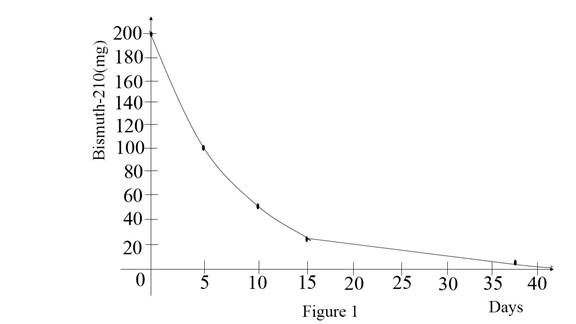# The amount remaining bismuth-210 after 15 days.### Single Variable Calculus: Concepts...

4th Edition
James Stewart
Publisher: Cengage Learning
ISBN: 9781337687805### Single Variable Calculus: Concepts...

4th Edition
James Stewart
Publisher: Cengage Learning
ISBN: 9781337687805

#### Solutions

Chapter 1.5, Problem 31E

(a)

To determine

## To find: The amount remaining bismuth-210 after 15 days.

Expert Solution

The amount remaining bismuth-210 after 15 days is 25mg.

### Explanation of Solution

Given:

The half-life of bismuth-210 is 5 days.

The mass of sample bismuth-210 = 200 mg.

Calculation:

Let m0 be the initial mass of bismuth-210.

That is m0=200mg .

After 5 days the bismuth level is m1=12m0

Substitute 200mg for m0 in m1 ,

m1=12(200)m1=100mg

After 10 days the bismuth level is m2=12m1

Substitute 100mg for m1 in m2 ,

m2=12(100)m2=50mg

After 15 days the bismuth level is m3=12m2

Substitute 50mg for m2 in m3 ,

m3=12(50)m3=25mg

Therefore, the amount of bismuth after 15 days is 25mg.

(b)

To determine

### To find: The amount of bismuth after t days.

Expert Solution

After t days mass of bismuth is mt=2t5(200) .

### Explanation of Solution

Calculation:

Consider half –life bismuth mass in 5 days = 12m0

Where m0=200mg .

After t=5xdays .where x be variable.

Therefore after t days mass of bismuth as follows,

mt=12x(m0) (1)

Substitute t5 for x and 200 for m0 in equation (1),

mt=12t5(200)mt=2t5(200)

Therefore, after t days mass of bismuth is mt=2t5(200) .

(c)

To determine

### To estimate: The remaining amount of bismuth after 3 weeks.

Expert Solution

After 3 weeks the amount of bismuth is m21=10.8818mg .

### Explanation of Solution

Given:

Consider after 5 days the amount of bismuth = 100 mg.

m0=200mg .

Calculation:

After 5 days the amount of bismuth is m1=12m0

By using part (b) solution mt=2t5(200) for t days.

Obtain the amount after 3 weeks that means 21 days.

Substitute 21 for t in mt=2t5(200) ,

m21=2215(200)m21=24.2(200)m21=0.0544(200)m21=10.8818mg

Therefore, after 3 weeks the amount of bismuth is m21=10.8818mg .

(d)

To determine

### To estimate: The amount of bismuth reduced to 1mg.

Expert Solution

The amount of bismuth mass reduced to 1mg for 38.2215 days.

### Explanation of Solution

Given:

The reduced amount of bismuth = 1mg.

Graph:

Let x axis for days and y axis for bismuth (mg).

Using online graphing calculator to draw the graphs for bismuth level and days as shown below Figure 1.Observe the graph, an initial stage bismuth mass is 200 mg.

Then after 5 days the mass is reducing to 100 mg.

After 10 the mass is reducing to 50 mg.

After 15 days mass is reducing to 25 mg.

Therefore, the mass of bismuth is reducing when the days are increasing.

Calculation:

From the graph, the general form of bismuth after t days mt=2t5(200) .

Substitute 1 for mt in mt=2t5(200) ,

1=2t5(200)1200=2t50.005=2t5

Take naturel logarithm on both sides,

ln(0.005)=ln(2t5)ln(0.005)=t5ln(2)ln(0.005)ln(2)=t5t=5(ln(0.005)ln(2))

Simplify further,

t=5(5.29830.6931)t=5(7.6443)t=38.2215

Therefore, the amount of bismuth mass reduced to 1mg for 38.2215 days.

### Have a homework question?

Subscribe to bartleby learn! Ask subject matter experts 30 homework questions each month. Plus, you’ll have access to millions of step-by-step textbook answers!